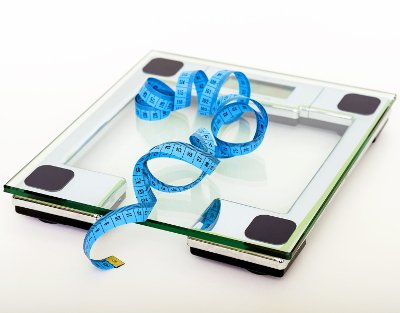# Body Surface Area (BSA) Calculator

Share:

Online BSA (Body Surface Area) calculator. Body surface area is used for several clinical purpose including calculation of dosage of medicines.

Enter your height and weight. Then click the "Calculate BSA" button.

*
ft
Feet
in
Inches
cm
Centimeters
*
lb
Pounds
kg
Kilograms
*

m2

Similar Fitness Calculators

## Body Surface Area (BSA) CalculatorThis on-line BSA calculator takes your height and weight and calculates the body surface area in m2. You have the option to select the formula you want the BSA to be calculated.

### Using the BSA calculator

1. Enter your Height either in centimeters or in feet.
2. Enter your Weight either in kilograms or pounds.
3. Then, click the Calculate BSA button. The body surface area will be calculated and the result will be displayed in the box below the button.

## Body Surface Area (BSA)

There are several formulas to calculate human body surface area. The popular once are Du Bois and Mosteller. Body surface area is mainly used for clinical purposes like medicine dosage calculations, etc...

### Formulas To Calculate Body Surface Area

#### 1. Du Bois Formula:

BSA = Wt0.425 x Ht0.725 x 0.007184

#### 2. Mosteller Formula:

BSA = Wt0.5 x Ht0.5 x 0.016667

#### 3. Haycock Formula:

BSA = Wt0.5378 x Ht0.3964 x 0.024265

#### 4. Gehan and George Formula:

BSA = Wt0.51456 x Ht0.42246 x 0.0235

#### 5. Boyd Formula:

BSA = Wt(0.6157 - 0.0188 log10 Wt) x Ht0.3 x 0.0333

#### 6. Fujimoto Formula:

BSA = Wt0.444 x Ht0.663 x 0.008883

#### 7. Takahira Formula:

BSA = Wt0.425 x Ht0.725 x 0.007241

#### 8. Schlich Formula

BSA for Women= Wt0.46 x Ht1.08 x 0.000975482

BSA for Men= Wt0.38 x Ht1.24 x 0.000579479

For all the above formulas:

• BSA = Body Surface Area in m2.
• Ht = Height in Meters (m).
• Wt = Weight in kilograms (Kg).